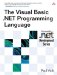# Financial Module

 <  Day Day Up  >

The Financial module contains functions that perform useful financial calculations.

## Historical

It may seem odd that the Visual Basic .NET runtime library contains financial functions, since the .NET Framework in general does not provide specialized financial functionality. Although the history is somewhat unclear, the financial functions are most likely a legacy of Visual Basic's use as Microsoft Excel's scripting language.

` Public Function DDB(ByVal Cost As Double, ByVal Salvage As Double, _   ByVal Life As Double, ByVal Period As Double, _   Optional ByVal Factor As Double = 2.0) As Double `

The DDB function calculates the depreciation of an asset for a specific time period using the double-declining balance method.

` Public Function FV(ByVal Rate As Double, ByVal NPer As Double, _   ByVal Pmt As Double, Optional ByVal PV As Double = 0, _   Optional ByVal Due As DueDate = DueDate.EndOfPeriod) As Double `

The FV function calculates the future value of an annuity based on periodic, fixed payments and a fixed interest rate.

` Public Function IPmt(ByVal Rate As Double, ByVal Per As Double, _   ByVal NPer As Double, ByVal PV As Double, _   Optional ByVal FV As Double = 0, _   Optional ByVal Due As DueDate = DueDate.EndOfPeriod) As Double `

The IPmt function calculates the interest payment for a given period of an annuity based on periodic, fixed payments and a fixed interest rate.

` Public Function IRR(ByRef ValueArray() As Double, _   Optional ByVal Guess As Double = 0.1) As Double `

The IRR function calculates the internal rate of return for a series of periodic cash flows (payments and receipts).

` Public Function MIRR(ByRef ValueArray() As Double, _   ByVal FinanceRate As Double, ByVal ReinvestRate As Double) As Double `

The MIRR function calculates the modified internal rate of return for a series of periodic cash flows (payments and receipts).

` Public Function NPer(ByVal Rate As Double, ByVal Pmt As Double, _   ByVal PV As Double, Optional ByVal FV As Double = 0, _   Optional ByVal Due As DueDate = DueDate.EndOfPeriod) As Double `

The NPer function calculates the number of periods for an annuity based on periodic, fixed payments and a fixed interest rate.

` Public Function NPV(ByVal Rate As Double, _   ByRef ValueArray() As Double) As Double `

The NPV function calculates the net present value of an investment based on a series of periodic cash flows (payments and receipts) and a discount rate.

` Public Function Pmt(ByVal Rate As Double, ByVal NPer As Double, _   ByVal PV As Double, Optional ByVal FV As Double = 0, _   Optional ByVal Due As DueDate = DueDate.EndOfPeriod) As Double `

The Pmt function calculates the payment for an annuity based on periodic, fixed payments and a fixed interest rate.

` Public Function PPmt(ByVal Rate As Double, ByVal Per As Double, _   ByVal NPer As Double, ByVal PV As Double, _   Optional ByVal FV As Double = 0, _   Optional ByVal Due As DueDate = DueDate.EndOfPeriod) As Double `

The PPmt function calculates the principal payment for a given period of an annuity based on periodic, fixed payments and a fixed interest rate.

` Public Function PV(ByVal Rate As Double, ByVal NPer As Double, _   ByVal Pmt As Double, Optional ByVal FV As Double = 0, _   Optional ByVal Due As DueDate = DueDate.EndOfPeriod) As Double `

The PV function calculates the present value of an annuity based on periodic, fixed payments to be paid in the future and a fixed interest rate.

` Public Function Rate(ByVal NPer As Double, ByVal Pmt As Double, _   ByVal PV As Double, Optional ByVal FV As Double = 0, _   Optional ByVal Due As DueDate = DueDate.EndOfPeriod, _   Optional ByVal Guess As Double = 0.1) As Double `

The Rate function calculates the interest rate per period for an annuity.

` Public Function SLN(ByVal Cost As Double, ByVal Salvage As Double, _   ByVal Life As Double) As Double `

The SLN function calculates the straight-line depreciation of an asset for a single period.

` Public Function SYD(ByVal Cost As Double, ByVal Salvage As Double, _   ByVal Life As Double, ByVal Period As Double) As Double `

The SYD function calculates the sum-of- years digits depreciation of an asset for a specified period.

 <  Day Day Up  >The Visual Basic .NET Programming Language
ISBN: 0321169514
EAN: 2147483647
Year: 2004
Pages: 173
Authors: Paul Vick

Similar book on Amazon